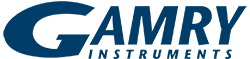# Gamry Simplex Fit- Algorithm Details

When fitting equivalent circuit models to Electrochemical Impedance Spectroscopy Data, Gamry utilizes a downhill simplex method to optimize the fit parameters.  The simplex method was first described by Nelder and Mead.  They described the simplex method as a method which adapts itself to the local landscape and contracts to a final minimum.  One advantage of the simplex method over other minimization techniques is that it does not require derivatives, just function evaluations.

Nelder, J. A. and Mead, R. "A Simplex Method for Function Minimization." Computer Journal 7, 308-313, 1965.

## Goodness of Fit

Goodness of fit is Chi Squared.  Chi squared is the calculated sum of the weighted residuals. Defined as the following:

ChiSq = Squared Sum of weighted ResidualsWeighting calculated as follows based on user input

Zmod weighting:Unity weighting:Custom weighting:Custom weighting can be used when weights are entered manually into the Zsig column of the data file using an ASCII editor.

## Residuals (Plot)

The residuals plot displayed is calculated as follows:## Error Margin Calculation

Errors are calculated using Maximum Likelihood Estimation.

We build an Information Matrix by Inverting the Hessian Matrix (a second order derivative matrix).

Next we calculate the variance-covariance matrix, and the standard errors are the square roots of the diagonal terms in the variance-covariance matrix.

×YES, WE ARE OPEN!

Gamry is accepting and shipping orders.  Our Technical Support and Sales are here to help.